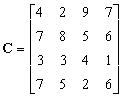1.Write Down the dual of the following prime problems

a. maxs. t.b. min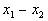s. t.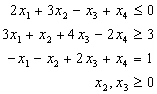c. max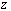s. t.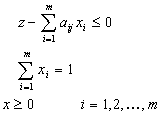2.Given the L.P.P

maxs.t.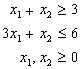Use duality theorem to prove that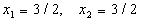is the optimal solution.

3.Find the optimal solution to the following L.P.Ps and to its dual.

a. max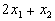s. t.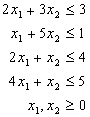b. min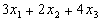s. t.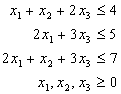c. mins. t.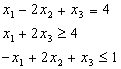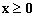4.Using WCS theorem find the optimal solution to the following L.P.Ps.

a. max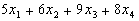s. t.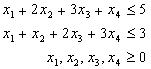b. max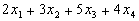s.t.c. min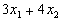s.t.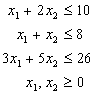5. Given the L.P.P

min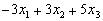s.t.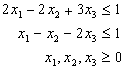the final tableau for this program (verify) isadd new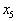with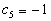and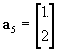Using the above tableau find the new optimal solution.

6. Given the L.P.P

min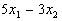s.t.solve the prime and its dual.

7. Given the L.P.P

max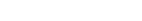s. t.find its dual then solve it Geometrically then solve the prime using WCS.

8. Use bland rule to solve the L.P.P.

min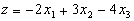s. t.add new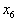withand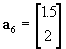Using the resulting tableau find the new optimal solution.

9. Solve

min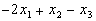s. t.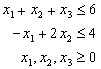find the possible changes in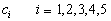while the solution remain optimal.

10. What the possible changes if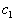changes from 1 to 2 to the L.P.P.

min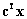s. t.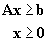with

,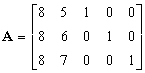,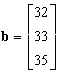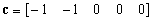11. Prove that the follows is optimal for Transportation problem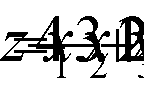a 4 14 18 24 24 2 4 6 b 6 14 28

then write the T.P in full form.

12. Solve the following Transportation problems

a.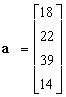,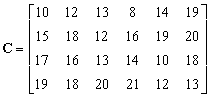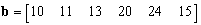b.c.

,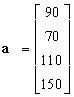,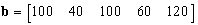d.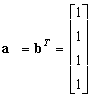,